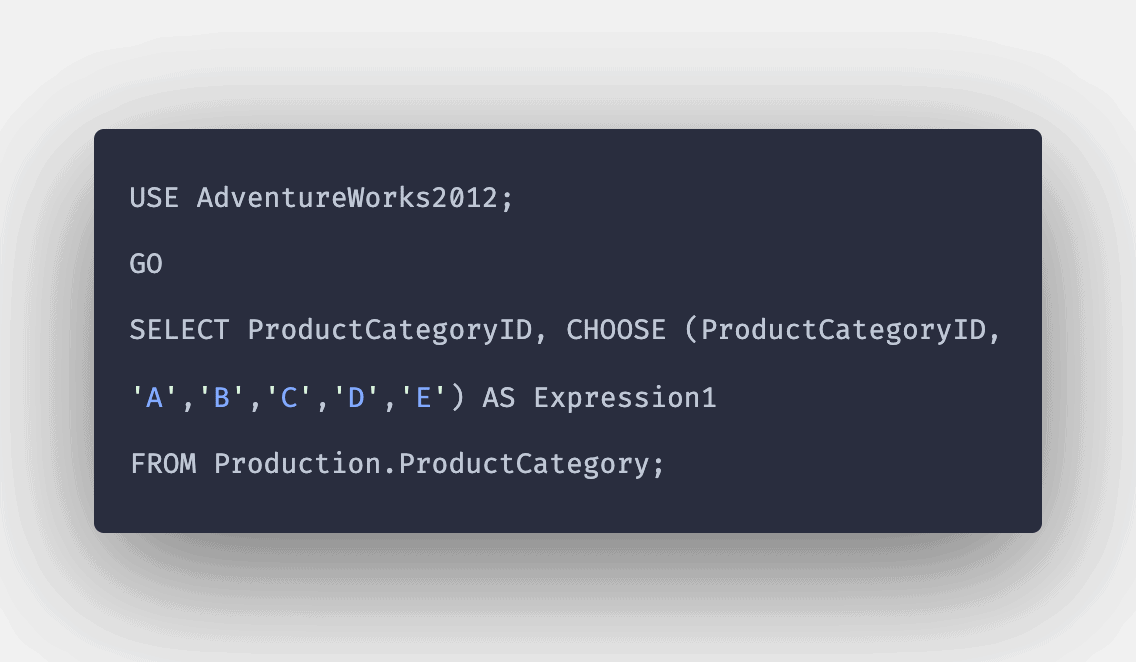# SQL CHOOSE() Function: Complete Guide

0
3SQL CHOOSE() function introduced in SQL Server 2012. This SQL Choose function returns the item at the specified index from the list of items.

## SQL CHOOSE

SQL CHOOSE() is a built-in function used to return an item from the list of items based on a specified index. The CHOOSE() function returns the item from a list of items at a specified index.

### Syntax

```CHOOSE (Index, Value1, Value2, ...., ValueN);
```

### Parameters

1. Index: It is an expression integer that specifies the index of the element to be returned. Here, indexing is based on 1-based.
2. Value1, Value2, …., ValueN: It denotes the list of items separated by commas.

#### Note

1. Value1 will be returned if the index specified is 1, and Value2 will be returned if the index specified is 2 and so on.
2. If the index is not in the integer format, then it will be type-casted to an integer.
3. If the index is out of bounds, then it will return NULL.

### Query 1

```Select CHOOSE (1, ‘App’, ‘Dividend’, ‘Com’);
```

```App
```

#### Explanation

The index specified was 1, and the App was indexed at the first position. So, the function returned the App as the output.

### Query 2

```Select CHOOSE (0, ‘App’, ‘Dividend’, ‘Com’);
```

```NULL
```

#### Explanation

Here, the index specified was 0, and as it was out of bounds, so the NULL value was returned.

### Query 3

```Select CHOOSE (2.3, ‘App’, ‘Dividend’, ‘Com’);
```

#### Output

```Dividend
```

#### Explanation

Here, an index that was specified at decimal representation was type-casted to an integer data type, which rounded off the value to 2. So, Dividend, which was indexed at 2nd position, was returned.

### Query 4

```Select CHOOSE (‘3’, ‘App’, ‘Dividend’, ‘Com’);
```

`Com`

#### Explanation

Here, an index that was specified at varchar was type-casted to the integer data type. So, Com, which was indexed at 3rd position, was returned.

That’s it for this tutorial.